Home Practice
For learners and parents For teachers and schools
Textbooks
Mathematics
JSS 1 JSS 2 JSS 3
Full catalogue
Pricing SupportLog in

We think you are located in Nigeria. Is this correct?

# Test yourself now

High marks in science are the key to your success and future plans. Test yourself and learn more on Siyavula Practice.

# Chapter 15: Probability

## 15.1 The basics of probability

Alkana and her friends want to go to an outdoor music festival. They can choose between two music festivals, one in early September and one in late October. Because the festivals are outdoors, Alkana is worried about rain. To find out about the chance of rain for each festival, Alkana looks online to find out how much rain there was in her area for the last ten years. She looks up early September and late October. She finds out that there has been rain 8 times over the last 10 years in in early September, but only 3 times in late October. She now knows that the probability of rain in late October is far lower, she suggests to her friends that they attend the late October festival.

In the example above, we are talking about the probability of something happening. We often use the word "chance" for probability, as when Alkana finds out about the chance of rain for each festival.

probability Probability is a mathematical way of describing how likely an event is to occur.

Some things always happen, so we can say it is certain that they will happen.
For example, it is certain that Tuesday is followed by Wednesday.

Some things will never happen, so we can say they are impossible.
For example, it is impossible for Wednesday to be followed by Tuesday.

Some things are not certain, but are not impossible either. They may happen or they may not happen. We can say that they are unlikely or likely to happen.

Sometimes there is an equal chance or a 50% chance that one of two things could happen. For example, if you toss a coin it might land on heads, or it might land on tails.

These words that are used to describe different probabilities are shown on the probability scale below.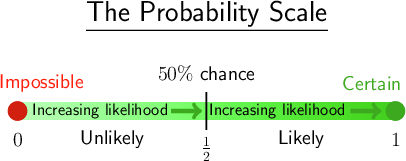We can also use numbers between 0 and 1 to describe the probability of an event happening:

• An event that is impossible is given a probability of 0.
• An event that is certain is given a probability of 1.
• All other probabilities on this scale are between 0 and 1.
• If there is a 50% chance, the fraction is $\frac{1}{2}$.
• The less likely an event is to happen, the smaller the fraction.
• The more likely an event is to happen, the larger the fraction.

### Worked example 15.1: Using words to describe probability

The diagram below shows the probability scale with an event, named $A$, positioned on the scale.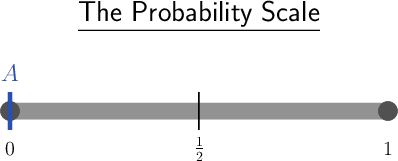What is the probability of event $A$ in words?

1. Step 1: Compare the probability scale showing $A$ with the scale showing the probabilities in words.In this question, event $A$ is placed at zero, meaning the event cannot happen. As shown on the probability scale, the event is impossible.

2. Step 2: Write a sentence giving the probability in words.

The probability of event $A$ in words is "impossible".

### Exercise 15.1: Use words to describe probability

1. Event $A$ is positioned on the probability scale shown in the diagram.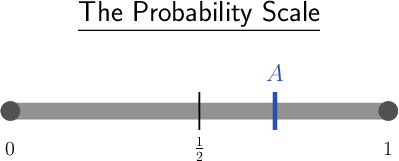In words, give the probability of event $A$.

Compare the probability scale showing $A$ with the scale showing the probabilities in words.In this question, $A$ is placed between $\frac{1}{2}$ and 1, so there is a good chance it will happen.

The probability of event $A$ in words is "likely".

2. The diagram below shows the probability scale with an event, named $A$, positioned on the scale.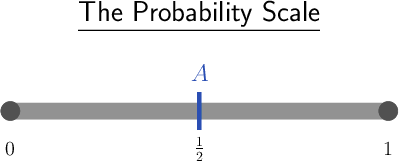What is the probability of event $A$?

Compare the probability scale showing $A$ with the scale showing the probabilities in words.In this question, $A$ is placed halfway along the line, meaning that the probability of event $A$ happening is 50%.

There is a 50% chance of event $A$ happening.

### Listing the outcomes of a probability experiment

We can use dice, coins and playing cards to do probability experiments. A probability experiment is any event that has a defined set of possible results or outcomes.

For example, all the possible outcomes of throwing a die are given in the set {1; 2; 3; 4; 5; 6}. All the possible outcomes of tossing a coin are given in the set {heads; tails}.

We have one die (singular) and many dice (plural).

Although a probability experiment has a defined set of possible outcomes, we don't know which one of these outcomes will take place each time. We perform an action, and record the outcome of the action each time.

probability experiment A probability experiment is an event that has a defined set of possible outcomes.

outcome An outcome is the possible result of an experiment.

Playing cards are often used to demonstrate probability experiments.

There are 52 cards in a pack of cards. These are divided into four suits: clubs, hearts, spades and diamonds.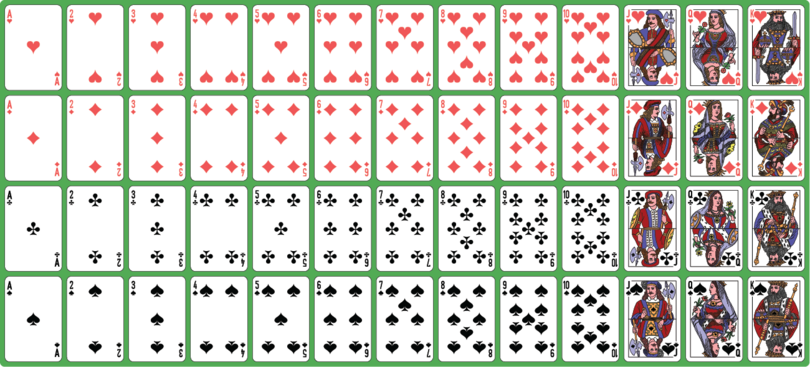In the illustration above:

• The red cards in the top row are the suit of hearts.
• The red cards in the second row are the suit of diamonds.
• The black cards in the third row are the suit of clubs.
• The black cards in the fourth row are the suit of spades.
• There are 13 cards in each suit.
• There are 26 black cards and 26 red cards in a pack of cards.
• There are 12 picture or face cards in a pack of cards (shown in the last three columns): four Jacks (J), four Queens (Q) and four Kings (K).
• There are four different Aces in a pack of cards, one for each suit. The Aces are shown in the first column.
• In each suit there are ten number cards. The Ace usually counts as the number 1, and then there are the cards numbered 2 to 10.
• There are therefore $4 \times 10 = 40$ number cards in a pack of cards.
• A pack of playing cards usually has two Joker cards, which are often removed for probability experiments.

### Worked example 15.2: Describing the possible outcomes of a probability experiment

Ganiru has a pack of cards with the Jokers removed. He takes one card from the pack of cards. How many possible outcomes are there for what card this could be?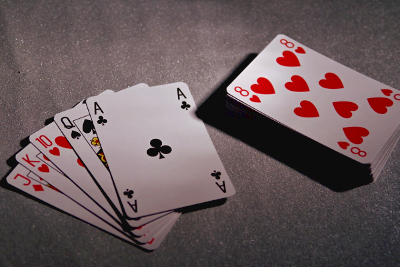1. Step 1: Remember how many cards there are in a pack of playing cards.

There are 52 cards in a pack of cards.

2. Step 2: Write down what this means for how many possible outcomes there are for this experiment.

When one card is taken from a pack of cards, it will be one of the 52 cards. There are 52 possible outcomes for this experiment.

### Exercise 15.2: List possible outcomes of an experiment

1. A coin is tossed.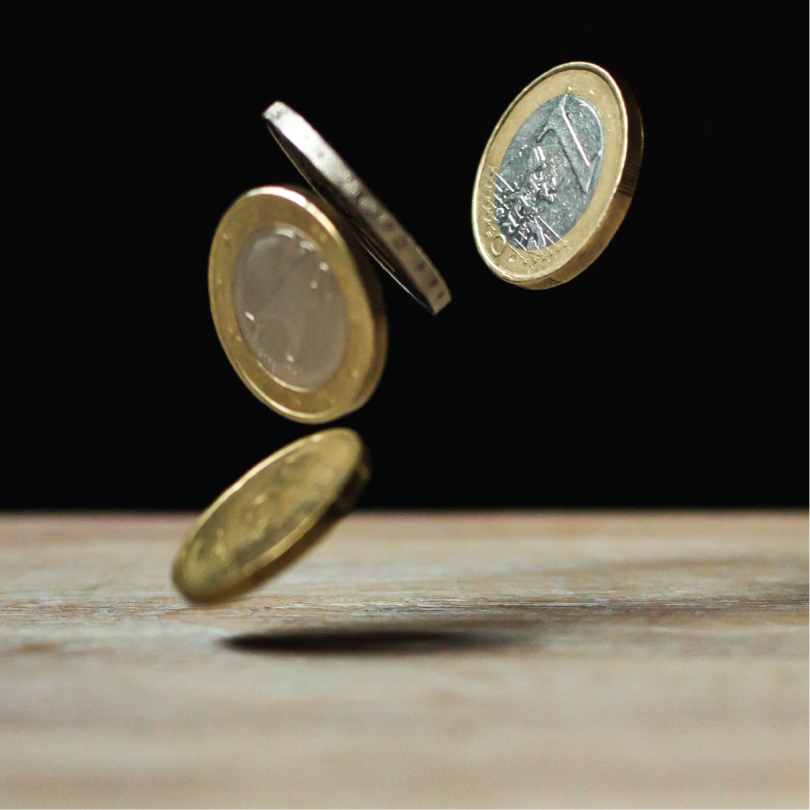1. Give the set of all the possible outcomes from this experiment.

When a coin is tossed, the set of all the possible outcomes is { $\text{heads; tails}$}.

1. How many possible outcomes are there for this experiment?

There are 2 possible outcomes for this experiment.

2. A die is thrown.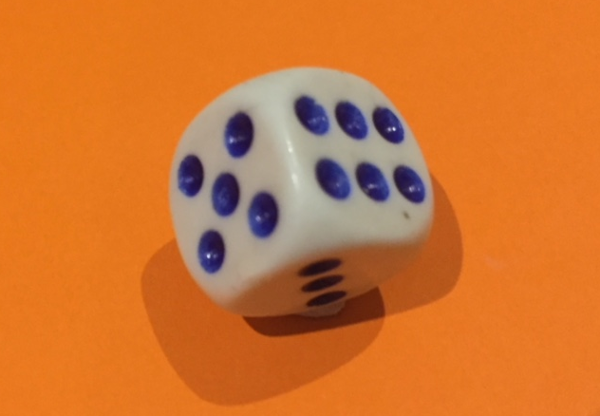1. Give the set of all the possible outcomes from this experiment.

When a die is thrown, the set of all the possible outcomes is { $\text{1; 2; 3; 4; 5; 6}$}.

1. How many possible outcomes are there for this experiment?

There are 6 possible outcomes for this experiment.

## 15.2 Calculating probabilities

Probability gives us a mathematical way of working out how likely it is that an event will happen. To do this, we first need to be very clear about the particular outcome that we are looking for.

For example, if we are flipping a coin, we might want to calculate the probability of the coin landing on tails. Then "landing on tails" is the outcome we are looking for. We call this the favourable outcome.

To calculate the probability of an event, we look at the ratio of the number of favourable outcomes to the number of possible outcomes. The number of possible outcomes is the total number of outcomes that is possible for one event.

When we flip a coin, we know that the number of possible outcomes is 2, because the set of all the possible outcomes is { $\text{heads; tails}$}.

If the favourable outcome is "landing on tails", there is 1 favourable outcome. There are 2 possible outcomes. So, the ratio of the number of favourable outcomes to the number of possible outcomes is 1 : 2.

You have learnt that a ratio can be written as a fraction, so this ratio can also be written as $\dfrac{1}{2}$.

favourable outcome The favourable outcome is the particular outcome that we are looking for.

possible outcomes The total number of outcomes that is possible for one event is the possible outcomes.

To calculate the probability of an event happening, we can use this formula:

$\text{Probability of an event}$ = $$\dfrac{\text{number of favourable outcomes}}{\text{number of possible outcomes}}$$

We can only use this formula to work out probability when all the outcomes of an activity are equally likely. For example, when we flip a coin, it is equally likely that we will get a heads or a tails, or when we toss a die, it is equally likely that we will get a 1, 2, 3, 4, 5 or 6.

To use this formula to calculate the probability of an event, we need to:

• find the number of possible outcomes
• find the number of favourable outcomes
• express the ratio as a fraction
• or we can divide the number of favourable outcomes by the number of possible outcomes in the fraction to get a decimal fraction.

### Using shapes to calculate probabilities

We can investigate probabilities using a group of shapes. For the probability experiment, we will assume that the group of shapes is in a bag, so the person picking out a shape cannot see the shapes. The person will pick out one shape at a time.

We will calculate the probability of selecting or picking one shape from a group of shapes. To do this, we need to know:

• the total number of shapes we are picking from
• which shape we are choosing as the favourable outcome
• how many of the chosen shapes there are altogether in the bag.

When we have worked this out, we can substitute into the probability formula.

### Worked example 15.3: Calculating a probability

What is the probability of selecting a triangle from this group of shapes? Write the probability as a fraction.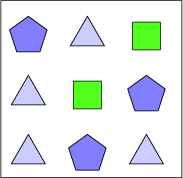1. Step 1: Write down the total number of possible outcomes.

There are 9 shapes, so the total number of possible outcomes is 9.

2. Step 2: Write down the number of favourable outcomes.

The favourable outcome is "selecting a triangle". There are 4 triangles, so the number of favourable outcomes is 4.

3. Step 3: Use the formula to calculate the probability of selecting a triangle from the nine shapes.

$\text{Probability}$ = $$\dfrac{\text{number of favourable outcomes}}{\text{number of possible outcomes}} = \dfrac{\text{4}}{\text{9}}$$

The probability of selecting a triangle is $\frac{4}{9}$.

### Exercise 15.3: Calculate probabilities

1. What is the probability of selecting a circle from the shapes below?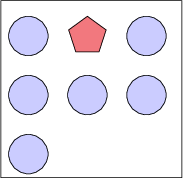Write the probability as a fraction.

There are 7 shapes, so the total number of possible outcomes = 7

There are 6 circles, so the number of favourable outcomes = 6.

$\text{Probability}$ = $$\dfrac{\text{number of favourable outcomes}}{\text{number of possible outcomes}} = \dfrac{\text{6}}{\text{7}}$$

The probability of selecting a circle is $\frac{6}{7}$.

2. What is the probability of selecting a circle from the shapes below?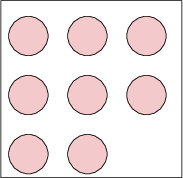Write the probability in its simplest form.

There are 8 shapes, so the total number of possible outcomes = 8

There are 8 circles, so the number of favourable outcomes = 8.

$\text{Probability}$ = $$\dfrac{\text{number of favourable outcomes}}{\text{number of possible outcomes}} = \dfrac{\text{8}}{\text{8}}$$ = 1

The probability of selecting a circle is 1.

### Using dice, coins and playing cards to calculate probabilities

When we do probability experiments using dice and coins, we assume that we have a fair dice and a fair coin.

• When a fair die is thrown, each of the numbers on the die is equally likely to occur.
• When a fair coin is flipped, the heads and the tails are equally likely to occur.

In a fair die, all the edges and corners are exactly the same, and the mass is evenly spread through the die. If the edges and corners were rubbed in different ways, or if there was a weight inside the die, the die would tend to fall on one number more than the others. This would be an unfair die.

A coin that lands on tails more often than on heads, because it is weighted or shaped in some way, would be an unfair coin.

### Worked example 15.4: Calculating probabilities using a die

Adebankole throws a fair die. Work out the probabilities listed below. Give your answers in their simplest form.

1. What is the probability that she gets a five?

2. What is the probability that she gets an even number?

3. What is the probability that she gets a seven?

4. What is the probability that she gets more than two?

5. What is the probability that she gets less than ten?

6. What is the probability that she gets a one or a six?

1. Step 1: Write down the total number of possible outcomes.

The possible outcomes are { $\text{1; 2; 3; 4; 5; 6}$}.

The total number of possible outcomes is 6.

2. Step 2: The favourable outcome is "get a five". Determine the probability of getting a five.

The number of favourable outcomes is 1.

$\text{Probability of getting a five}$ = $$\dfrac{\text{number of favourable outcomes}}{\text{number of possible outcomes}} = \dfrac{\text{1}}{\text{6}}$$

3. Step 3: The favourable outcome is "get an even number". Determine the probability of getting an even number.

The favourable outcomes are { $\text{2; 4; 6}$}.

The number of favourable outcomes is 3.

$\text{Probability of getting an even number}$ = $$\dfrac{\text{number of favourable outcomes}}{\text{number of possible outcomes}} = \dfrac{\text{3}}{\text{6}} = \dfrac{\text{1}}{\text{2}}$$

4. Step 4: The favourable outcome is "get a seven". Determine the probability of getting a seven.

There are no favourable outcomes, because none of the numbers on a die is a seven.

The number of favourable outcomes is 0.

$\text{Probability of getting a seven}$ = $$\dfrac{\text{number of favourable outcomes}}{\text{number of possible outcomes}} = \dfrac{\text{0}}{\text{6}}$$ = 0

You could get to this answer without doing a calculation, because, using the probability scale and your own logic, if the favourable outcome is not possible, then the probability is 0. But if you are asked to calculate a probability, you should always do the calculation, as marks will be given for the calculation.

5. Step 5: The favourable outcome is "get a number more than two". Determine the probability of getting a number more than two.

The favourable outcomes are { $\text{3; 4; 5; 6}$}.

The number of favourable outcomes is 4.

$\text{Probability of getting a number more than two}$ = $$\dfrac{\text{number of favourable outcomes}}{\text{number of possible outcomes}} = \dfrac{\text{4}}{\text{6}} = \dfrac{\text{2}}{\text{3}}$$

6. Step 6: The favourable outcome is "get a number less than ten". Determine the probability of getting a number less than ten.

All the outcomes are favourable as all the numbers are less than ten.

The number of favourable outcomes is 6.

$\text{Probability of getting a number less than ten}$ = $$\dfrac{\text{number of favourable outcomes}}{\text{number of possible outcomes}} = \dfrac{\text{6}}{\text{6}}$$ = 1

7. Step 7: The favourable outcome is "get a one or a six". Determine the probability of getting a one or six.

The favourable outcomes are { $\text{1; 6}$}.

The number of favourable outcomes is 2.

$\text{Probability of getting a one or a six}$ = $$\dfrac{\text{number of favourable outcomes}}{\text{number of possible outcomes}} = \dfrac{\text{2}}{\text{6}} = \dfrac{\text{1}}{\text{3}}$$

### Exercise 15.4: Calculate probabilities using dice and cards

1. Osinachi throws a fair die.1. Determine the total number of possible outcomes.

The possible outcomes are { $\text{1; 2; 3; 4; 5; 6}$}.

Total number of possible outcomes = 6

1. What is the probability that he will get a 2?

The favourable outcome is a {2}.

Number of favourable outcomes = 1

$\text{Probability of getting a two}$ = $$\dfrac{\text{number of favourable outcomes}}{\text{number of possible outcomes}} = \dfrac{\text{1}}{\text{6}}$$

1. What is the probability that he will get an odd number? Give the answer as a fraction in simplified form.

The favourable outcomes are { $\text{1; 3; 5}$}.

Number of favourable outcomes = 3

$\text{Probability of getting an odd number}$ = $$\dfrac{\text{number of favourable outcomes}}{\text{number of possible outcomes}} = \dfrac{\text{3}}{\text{6}} = \dfrac{\text{1}}{\text{2}}$$

1. What is the probability that he will get a number bigger than four? Give the answer as a fraction in simplified form.

The favourable outcomes are { $\text{5; 6}$}.

Number of favourable outcomes = 2

$\text{Probability of getting a number bigger than four}$ = $$\dfrac{\text{number of favourable outcomes}}{\text{number of possible outcomes}} = \dfrac{\text{2}}{\text{6}} = \dfrac{\text{1}}{\text{3}}$$

2. Musa picks a card at random from an ordinary pack of cards.1. What is the total number of possible outcomes?

A pack of cards has of 52 cards, so the total number of possible outcomes is 52.

1. What is the probability that the card is the two of diamonds? Give the answer as a fraction in its simplest form.

An ordinary pack of cards is made up of four different suits of cards, each one consisting of thirteen cards. There is only one two of diamonds in a pack of cards, so the number of favourable outcomes is 1.

$\text{Probability of getting a two of diamonds}$ = $$\dfrac{\text{number of favourable outcomes}}{\text{number of possible outcomes}} = \dfrac{\text{1}}{\text{52}}$$

1. What is the probability that the card will be an ace (A)? Give the answer as a fraction in its simplest form.

There are four aces in a pack of cards, so the number of favourable outcomes is 4.

$\text{Probability of getting an ace}$ = $$\dfrac{\text{number of favourable outcomes}}{\text{number of possible outcomes}} = \dfrac{\text{4}}{\text{52}} = \dfrac{\text{1}}{\text{13}}$$

1. What is the probability that the card will be a red card or a black card? Give the answer as a fraction in its simplest form.

All the cards in a pack of cards are red or black so the number of favourable outcomes is 52.

$\text{Probability of getting a red card or a black card}$ = $$\dfrac{\text{number of favourable outcomes}}{\text{number of possible outcomes}} = \dfrac{\text{52}}{\text{52}}$$ = 1

1. What is the probability that the card will be a heart? Give the answer as a fraction in its simplest form.

There are thirteen hearts in a pack of cards, so the number of favourable outcomes is 13.

$\text{Probability of getting a heart}$ = $$\dfrac{\text{number of favourable outcomes}}{\text{number of possible outcomes}} = \dfrac{\text{13}}{\text{52}} = \dfrac{\text{1}}{\text{4}}$$

1. What is the probability that the card will be a picture card, which means a king (K), queen (Q) or jack (J)? Give the answer as a fraction in its simplest form.

There are four kings, four queens and four jacks in a pack of cards, so the number of favourable outcomes is 12.

$\text{Probability of getting a picture card}$ = $$\dfrac{\text{number of favourable outcomes}}{\text{number of possible outcomes}} = \dfrac{\text{12}}{\text{52}} = \dfrac{\text{3}}{\text{13}}$$

## 15.3 Practical applications

We can determine the probability of events occurring in everyday life.

An example of one practical application is to use the information of how students travel to school each day to find the probability that one of the students, chosen at random, uses a particular method to get to school every day.

Often the information we are working with is given in a frequency table, which means that it is easy to determine the values we need to calculate probabilities.

### Worked example 15.5: Calculating probabilities using frequency tables

A survey was done to find out how every student in JSS2 at one school usually travelled to school each day.

The information was summarised in a frequency table.

Method used to travel to school Number of students
Bus 15
Minibus taxi 15
Bicycle 25
Car 20

What is the probability that a student, chosen at random, rides a bicycle to school every day? Give your answer as a fraction in its simplified form.

1. Step 1: Determine the number of possible outcomes by adding up the number of JSS2 students.

Total number of JSS2 students = $\text{15 + 15 + 25 + 20 = 75}$

2. Step 2: Determine the number of favourable outcomes by finding the number of students that ride a bicycle to school.

From the table we can see that the number of students that ride a bicycle to school is 25.

3. Step 3: Determine the probability that a student, chosen at random, rides a bicycle to school by substituting into the formula.

$\text{Probability of a student riding a bicycle to school}$ = $$\dfrac{\text{number of students that ride a bicycle to school}}{\text{total number of students in JSS2}} = \dfrac{\text{25}}{\text{75}} = \dfrac{\text{1}}{\text{3}}$$

The probability of a student, chosen at random, riding a bicycle to school is $\frac{1}{3}$.

### Exercise 15.5: Calculate probabilities using frequency tables

1. The person running the school cafeteria recorded what lunches students bought from the school cafeteria on one day.

The information was summarised in a frequency table.

Lunch bought Number of students
Jollof and chicken 20
Plantain and beans 10
Okra soup and fufu 10
Rice and meatballs 15

What is the probability that a student, chosen at random, bought plantain and beans for lunch? Give your answer as a simplified fraction.

Total number of students that bought lunch = $\text{20 + 10 + 10 + 15 = 55}$

Number of students that bought plantain and beans = 10

$\text{Probability of a student buying plantain and beans}$ = $$\dfrac{\text{number of students that bought plantain and beans}}{\text{number of students that bought lunch}} = \dfrac{\text{10}}{\text{55}} = \dfrac{\text{2}}{\text{11}}$$

The probability that a student, chosen at random, bought plantain and beans is $\frac{2}{11}$.

2. A human resource manager at a firm wants to work out how the staff travel to and from work each day. She carries out a survey, and summarises the results in a frequency table.

Method of travelling to work Number of staff members
Lift in someone else's car 25
Train 15
Own car 15
Minibus taxi 10

What is the probability that a staff member, chosen at random, takes a lift in someone else's car every day? Give your answer as a fraction in simplified form.

Total number of staff members = $\text{25 + 15 + 15 + 10 = 65}$

Number of staff members that take a lift in someone else's car = 25

$\text{Probability of a staff member taking a lift}$ = $$\dfrac{\text{number of staff members that taking a lift}}{\text{Total number of staff members}} = \dfrac{\text{25}}{\text{65}} = \dfrac{\text{5}}{\text{13}}$$

The probability that a staff member, chosen at random, walks to work is $\frac{5}{13}$.

## 15.4 Summary

• Probability is a mathematical way of describing how likely an event is to occur.
• We can use words to describe the probability of something happening.
• If something always happens, we say it is certain.
• If something never happens, we say it is impossible.
• If something may or may not happen, we say it is likely, unlikely, or that there is a 50% or equal chance that it will happen.
• We can also use numbers from 0 to 1 to describe the probability of something happening.
• An event that is impossible is given a probability of 0.
• An event that is certain is given a probability of 1.
• All other probabilities are fractions between 0 and 1.
• When all the outcomes of a probability experiment are equally likely, we can calculate the probability of one of the events using this formula:

$\text{Probability of an event}$ = $$\dfrac{\text{number of favourable outcomes}}{\text{number of possible outcomes}}$$

• The favourable outcome is the particular outcome that we are looking for.
• The total number of outcomes that is possible for one event is the possible outcomes.